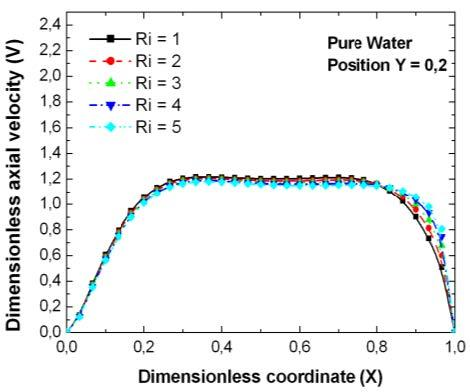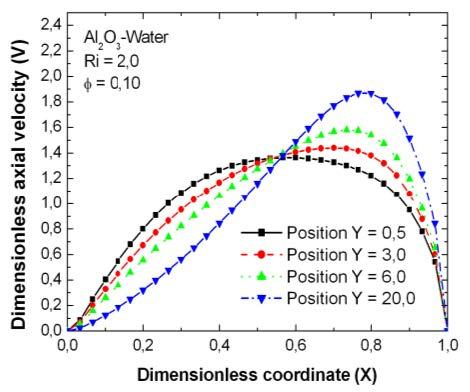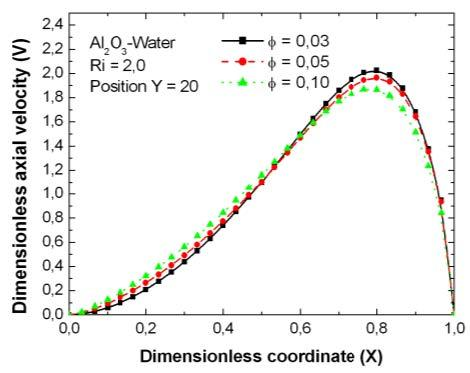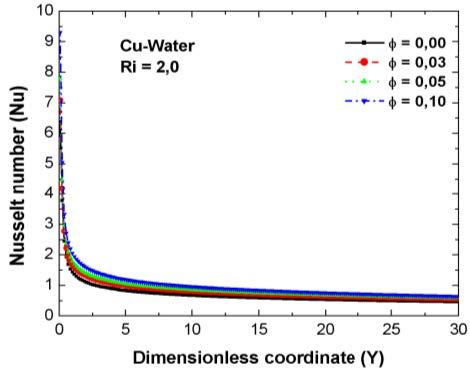# Numerical Investigation of Nanofluids Mixed Convection in a Vertical Channel

Numerical Investigation of Nanofluids Mixed Convection in a Vertical Channel
Département de Technologie, Faculté de Technologie, Université 20 Août 1955, Skikda 21000, Algérie

Département de Génie des transports, Université des frères Mentouri Constantine 1, Constantine 25000, Algérie

Laboratory of Mechanics, University Amar Telidji, Laghouat 03000, Algeria

Corresponding Author Email:
m.teggar@lagh-univ.dz
Page:
575-580
|
DOI:
https://doi.org/10.18280/mmep.060413
26 April 2019
|
Revised:
6 December 2019
|
Accepted:
13 December 2019
|
Available online:
24 December 2019
| Citation

OPEN ACCESS

Abstract:

In this paper, mixed convection of nanofluids is numerically investigated in an asymmetric heated vertical channel. Two nanofluids are considered: copper-water and alumina oxide-water. A single phase model is used with considering approximations of Brinkman and Maxwell-Garnetts for the nanofluids dynamic viscosity and the effective thermal conductivity, respectively. The non-dimensional conservation equations with along the boundary conditions are solved by using the finite volume method and SIMPLE algorithm. The effects of nanoparticle volume fraction (0-10%) on the flow patterns and heat transfer characteristics are analyzed and discussed. Results showed that nanoparticles volume fraction with assisted buoyancy force (induced by one heated channel wall) has a strong effect on nanofluid velocities and average Nusselt number. The Cu-water nanofluid showed better thermal performance than that of Al2O3-water.

Keywords:

mixed convection, nanofluid, vertical channel, volume fraction

1. Introduction

Mixed convection in vertical channels can be encountered in liquid based cooling systems such as automobile radiators, fuel cells and other thermal applications. Mixed convection of conventional fluids in vertical channels had received increasing attention. Therefore, earlier comprehensive works had been carried out to understand flow behavior and heat transfer characteristics between parallel plates [1-6].

Enhancement of coolant performances can help designing efficient thermal systems. The enhancement can be made by inserting metallic nanoparticles in conventional working fluids (nanofluids). Solid nanoparticles are known for their high thermal conductivity. In addition, the nano-scale particles can offer larger heat exchange and hence improve thermal properties of nanofluids. However, since the pioneer work of Choi and Eastman (1995) , nanofluids attract more and more investigators.

Solid concentration in nanofluid is one of the most influencing parameters on the fluid properties and heat transfer characteristics. The influence of nanoparticle concentration on thermal performance of nanofluids was investigated by many researchers. Sheikholeslami and Ganji  investigated thermal performance of copper nanoparticles in water as base fluid flowing between parallel plates. They reported that Nusselt number increases with increasing nanoparticle concentration. Fakour et al.  analyzed the effect of various thermal and dynamic parameters on heat transfer of a nanofluid in a vertical channel. They indicated that heat transfer was significantly improved by increasing nanoparticle concentration. Xu and Pop  studied analytically the effect of increasing different nanoparticles in water flowing between two heated parallel plates with increasing temperature along. Temperature and velocitiy distributions were analyzed. Assisting and opposing buoyancy effects were considered. The authors reported that heat transfer enhances with increasing nanoparticle concentration.

For forced convection, experimental and theoretical works confirmed heat transfer enhancement with increasing nanoparticle concentration . Nevertheless, this regular trend is not always observed in many experimental and numerical works [12-16]. Khanafer and Vafai discussed these contradictory findings in the literature .

Talebi et al.  reported that at low Rayleigh number, increasing Reynolds number resulted in negative effect of the particle concentration on heat transfer characteristics. The authors carried out a numerical investigation on Cu-water nanofluid in a lid-driven square cavity. The ranges of investigated parameters were Re=1-100 and Ra=104-106 for volume fractions up to 5 %.

However, mixed convection of nanofluids in vertical channels has not been sufficiently investigated especially for asymmetric heated walls.

The aim of this numerical study is to analyze the laminar mixed convection of two nanofluids developing in a vertical channel with one isothermally heated wall. Cu-water and Al2O3-water nanofluids are considered. Pure water is also investigated as baseline case in this study. The influence of solid particle volume fraction on the velocity distribution and Nusselt number are investigated for Re=100 and Ri=2.

2. Modelling

2.1 Physical model

Consider the two-dimensional laminar fluid in a vertical channel. The two parallel walls of height L are separated by a distance H. The y-axis is parallel to the gravitational acceleration vector while the x-axis is perpendicular to the walls. The origin of the x-axis is on the left hand (cooler) wall and the origin of the y-axis is at the entrance of the nanofluid (Figure 1). The left hand channel wall is kept at constant temperature T1 while the right hand (heated) wall of the channel is maintained at a constant temperature T2 (T2 > T1). The nanofluid inlet velocity is V0 at constant temperature (reference) T0 = T1. The nanofluids are supposed to be Newtonian and incompressible. The viscous dissipation is neglected. Boussinesq approximation is employed in this study.

## 1.jpgFigure 1. Sketch of the physical problem

2.2 Mathematical modeling

Single-phase model is considered in the study where the nanofluids are regarded as a continuous medium. Assuming that the nanoparticles are homogenously dispersed in the base fluid, the properties of the nanofluids can be calculated for the density:

$\rho_{n f}=(1-\phi) \rho_{f}+\phi \rho_{s}$    (1)

For the heat capacity:

$\left(\rho c_{p}\right)_{n f}=(1-\phi)\left(\rho c_{p}\right)_{f}+\phi\left(\rho c_{p}\right)_{s}$    (2)

For the thermal expansion coefficient:

$(\rho \beta)_{n f}=(1-\phi)(\rho \beta)_{f}+\phi(\rho \beta)_{s}$    (3)

For the thermal diffusivity:

$\alpha_{n f}=k_{n f} /\left(\rho C_{p}\right)_{n f}$     (4)

The viscosity is approximate by Brinkman model :

$\mu_{n f}=\mu_{f} /(1-\phi)^{2,5}$      (5)

The effective thermal conductivity is approximated by Maxwell-Garnetts model :

$\frac{k_{n f}}{k_{f}}=\frac{k_{s}+2 k_{f}-2 \phi\left(k_{f}-k_{s}\right)}{k_{s}+2 k_{f}+\phi\left(k_{f}-k_{s}\right)}$     (6)

The non-dimensional governing equations of the two-dimensional flow and heat transfer in Cartesian coordinates are given by:

$\frac{\partial U}{\partial X}+\frac{\partial V}{\partial Y}=0$      (7)

$U \frac{\partial U}{\partial X}+V \frac{\partial U}{\partial Y}=-\frac{\partial P}{\partial X}+\frac{1}{R e} \frac{\rho_{f}}{\rho_{n f}} \frac{1}{(1-\phi)^{2,5}}\left[\frac{\partial^{2} U}{\partial X^{2}}+\frac{\partial^{2} U}{\partial Y^{2}}\right]$     (8)

\begin{aligned} U \frac{\partial V}{\partial X}+V \frac{\partial V}{\partial Y}=-\frac{\partial P}{\partial Y} &+\frac{1}{R e} \frac{\rho_{f}}{\rho_{n f}} \frac{1}{(1-\phi)^{2,5}}\left[\frac{\partial^{2} V}{\partial X^{2}}+\frac{\partial^{2} V}{\partial Y^{2}}\right]+\frac{(\rho \beta)_{n f}}{\rho_{n f} \beta_{f}} R i \theta \end{aligned}      (9)

$U \frac{\partial \theta}{\partial x}+V \frac{\partial \theta}{\partial Y}=\frac{a_{n f}}{a_{f}} \frac{1}{R e P r}\left(\frac{\partial^{2} \theta}{\partial x^{2}}+\frac{\partial^{2} \theta}{\partial Y^{2}}\right)$     (10)

where, Pr, Re and Ri denote Prandtl number, Reynolds number and Richardson number, respectively. They are defined as follows:

$\operatorname{Pr}=v_{f} / a_{f} ; \operatorname{Re}=V_{0} H / v_{f} ; R i=g \beta_{f}\left(T_{0}-T_{w}\right) H / V_{0}^{2}$

The dimensionless variables of the above equations are defined as follows:

\begin{aligned} X=& x / H ; Y=y / H ; U=u / V_{0} ; \mathrm{V}=v / V_{0} \\ \theta=&\left(T-T_{0}\right) /\left(T_{2}-T_{0}\right) ; P=p / \rho_{n f} V_{0}^{2} \end{aligned}

The following boundary conditions are considered:

At the channel inlet:

$U=0, V=1$ and $\theta=0$     (11-a)

At the channel outlet:

$\frac{\partial^{2} U}{\partial Y^{2}}=\frac{\partial^{2} V}{\partial Y^{2}}=\frac{\partial^{2} \theta}{\partial Y^{2}}=0$    (11-b)

On the left hand wall:

$U=0, V=0$ and $\theta=0$     (11-c)

On the right hand wall:

$U=0, V=0$ and $\theta=1$      (11-d)

The local and average Nusselt numbers are defined as follows:

$N u=-\frac{k_{n f}}{k_{f}}\left(\frac{\partial \theta}{\partial x}\right)_{X=1} ; \overline{N u}=\frac{1}{L} \int_{0}^{L} N u d Y$     (12)

2.3 Numerical procedure

In this investigation, discretization of the governing differential equations is based on the finite volume approach. The velocity – pressure coupling is solved by the SIMPLE procedure . The algebraic equations are solved by using the Thomas algorithm. The numerical predictions of the present model had been previously validated by the author of the present study Kholai . Comparisons had been done with experimental and numerical results available in the literature.

3. Results and Discussion

The numerical results were obtained for Reynolds number Re=100, Richardson number range: Ri=1 to 5 while the nanoparticle volume fractions ranged from 0.00 to 0.10.

The thermophysical properties of the base fluid (water) and nanoparticles are presented in Table 1.

Table 1. Thermophysical properties of water and nanoparticles (T = 300K)

 Water Cu Al2O3 $\rho$ (kg.m-3) 997.1 8933 3970 $C_{P}$ (J.kg-1.K-1) 4179 385 765 k (W.m-1.K-1) 0,613 401 36 $\beta \times 10^{5}$ (K-1) 27,6 1,67 0.85 $\mu$ (Pa.s) 855 ×10-6 - -

## 2a.jpg(a)

## 2b.jpg(b)

## 2c.jpg(c)

Figure 2. Effect of the Richardson number on the axial velocity profiles at different positions for pure water

Figure 2 shows the effect of Richardson number on the axial velocity profiles of pure water at different positions along the channel. At the channel entrance (Y = 0.2), the effect of Richardson number is negligible. But when the fluid moves up through the channel, it is found that the flow in the vicinity of the hot wall is accelerated with increasing Richardson number.

The velocity profile presents a crest in this buoyancy affected region. This result can be explained by the appearance of natural convection, which increases the velocity of fluid particles in the vicinity of the wall. These results are consistent with analytical results presented in previous works [3-5].

## 3.jpgFigure 3. Effect of the Richardson number on the axial development of the Nusselt number for pure water

## 4a.jpg(a)

## 4b.jpg(b)

Figure 4. Axial development of the axial velocity for two nanofluids: (a) Cu-water; (b) Al2O3–water

The effect of Richardson number on the axial development of Nusselt number of pure water is illustrated in Figure 3. One can observe that the effect of Richardson number is negligible at the entrance region of the channel. As the fluid advances in the channel, we observe that the increase in Richardson number increases the Nusselt number and hence promotes heat transfer between the fluid and the heated channel wall.

The axial development of the nanofluids axial velocity profiles for ø=10 vol % and Ri = 2.0 are depicted in Figure 4. It can be noticed that both nanofluids show quite similar developments of flow profiles. This means that the type of nanoparticles has no significant effect on the velocity profile at the entrance region of the channel. At Y = 0.5, the axial velocity profile is symmetric with respect to the channel centerline. As moving away from the channel entrance, the velocity profile is distorted. The velocities enlarge continuously in the right hand half region of the channel with an increasing crest. The crest moves towards the heated channel as the flow develops in the channel. Meanwhile, the velocities diminish in the left half of the channel towards the cooler wall which is the consequence of mass conservation constrains. Unlike the cooler wall where the viscous forces tend to retard the velocities near the wall, the velocities near the hot wall are enhanced as can be seen on the two Figures (4-a) and (4-b) corresponding respectively to copper and aluminum oxide nanofluids. This enhancement is attributed to the assisting buoyancy force that allows the nanofluid to overcome the negative effect of the viscous boundary layer on the flow velocity.

Figure 5 shows the effect of the type of nanoparticles on the axial evolution of Nusselt number for Ri = 2.0. The solid volume fractions are set 10 % for the two nanofluids (Cu–water and Al2O3-water). The two nanofluids have similar behavior. Nusselt numbers decrease substantially at the channel entrance for the three fluids. On the fully developed flow region, Nusselt number has asymptotic trend. At a given axial position, it can be seen that larger nanoparticle volume fractions correspond to higher Nusselt numbers. Improved convective heat transfer is obtained by increasing solid volume fraction. Thus, adding metallic nanoparticles (Cu/Al2O3) of high thermal conductivity results in increasing of the nanofluid effective thermal conductivity.

## 5.jpgFigure 5. Effect of the type of nanoparticles on the axial evolution of the Nusselt number

The axial velocity component profile of the two nanofluids Cu-water and Al2O3–water at Y = 20 for Ri = 2 and for different nanoparticle volume fractions (0. 03; 0.05 and 0.10) are plotted in Figure 6. First, similar trends can be observed in the two figures (6-a) and (6-b) corresponding respectively to Cu-water and Al2O3-water nanofluids. Increasing the nanoparticle volume fraction leads to a decrease in the velocities at the vicinity of the heated wall. Besides, the unique crest of the velocity profile moves slightly towards the channel center as the nanoparticle volume fraction is augmented. The crest amplitude decreases with increasing the nanoparticles volume fraction. This can be explained by the effect of adding nanoparticles in the nanofluid; which results in increasing viscous forces.

In Figure 7 is illustrated the axial evolution of the Nusselt number for Ri = 2. Nusselt number decreases substantially at the channel entrance and then asymptotes at fully developed flow region. At a given channel axial position, it can be seen that higher volume fraction corresponds to higher convective heat transfer. This is due to improvement of the effective thermal conductivity caused by adding solid nanoparticles (Cu or Al2O3) of high thermal conductivity. These results are consistent with other findings of the literature [8-10].

## 6a.jpg(a)

## 6b.jpg(b)

Figure 6. Effect of nanoparticle volume fraction on the axial velocity (a) Cu-water; (b) Al2O3-water

## 7a.jpg(a)

## 7b.jpg(b)

Figure 7. Effect of the nanoparticles volume fraction (ø) on the axial evolution of the Nusselt number for two nanofluids: (a) Cu-water; (b) Al2O3-water

Figure 8 show the average Nusselt number as function of the nanoparticle volume fraction (ø) of the two considered nanofluids for Ri = 2. Increasing the nanoparticle volume fraction increases the average Nusselt number in the two cases (i. e. adding Cu and Al2O3). This fact is due to the higher effective thermal conductivity of the nanofluids. For a given nanofluid, Cu-water shows higher heat transfer rate than that of Al2O3-water. This effect is due to the higher thermal conductivity of copper compared to Al2O3. Improvement of heat transfer characteristics can be obtained with Cu nanoparticles rather than those of Al2O3

## 8.jpgFigure 8. Effect of the nanoparticles volume fraction (ø) on the average Nusselt number for two nanofluids

4. Conclusions

Numerical simulations have been carried out on mixed convection of two nanofluids (Cu-water and Al2O3-water) flowing upwardly in an asymmetric heated vertical channel. The effect of nanoparticle volume fraction (0≤Φ≤10 vol %) has been investigated for Re=100 and Ri=2. Results have showed that:

• The nanoparticle type did not affect the axial velocity profile.
• Increasing solid volume fraction resulted in a decrease in the axial velocities in the buoyancy region.
• The velocities profile crest caused by the buoyant force diminished in amplitude with increasing particle volume fraction.
• Both nanoparticles enhanced heat transfer of the nanofluids
• Copper nanoparticles showed larger heat transfer than that of alumina oxide

Finally, apparition of the reversed flow and its conditions can be considered for future research.

Nomenclature
 $a$ thermal diffusivity, m2.s-1 Cp speciﬁc heat at constant pressure, J.kg−1.K−1 g gravitational acceleration, m.s−2 Gr Grashof number k thermal conductivity, W.m−1.K−1 H channel width, m L channel length , m Nu Nusselt number $\overline{N u}$ average Nusselt number p pressure, Pa P dimensionless pressure Pr Prandtl number Re Reynolds number Ri Richardson number T Tmperature, K U, V dimensionless velocity components u, v velocity components, m x, y cartesian coordinates, m X,Y dimensionless coordinates Greek symbols β thermal expansion coefficient, K−1 θ dimensionless temperature ν kinematic viscosity, m2.s−1 μ dynamic viscosity, Kg. m-1.s−1 ρ density, kg.m−3 ø nanoparticles volume fraction Subscripts 1 left wall 2 rigth wall 0 inlet conditions nf nanofluid f base fluid s solid particles w value at the wall 1 left wall 2 rigth wall
References

 Beckett, P.M. (1980). Combined natural and forced-convection between parallel vertical walls. SIAM Journal on Applied Mathematics, 39(2): 372-384. https://doi.org/10.1137/0139031

 Beckett, P.M., Friend, I.E. (1984). Combined natural and forced convection between parallel walls: developing flow at higher Rayleigh numbers. International Journal of Heat and Mass Transfer, 27(4): 611-621. https://doi.org/10.1016/0017-9310(84)90033-4

 Cheng, C.H., Kou, H.S., Huang, W.H. (1990). Flow reversal and heat transfer of fully developed mixed convection in vertical channels. Journal of Thermophysics, 4(3): 375-383. https://doi.org/10.2514/3.190

 Hamadah, T.T., Wirtz, R.A. (1991). Analysis of laminar fully developed mixed convection in a vertical channel with opposing buoyancy. ASME Journal of Heat Transfer, 113(2): 507-510. https://doi.org/10.1115/1.2910593

 Barletta, A. (1991). Analysis of combined forced and free flow in a vertical channel with viscous dissipation and isothermal-isoflux boundary conditions. ASME Journal of Heat Transfer, 121(2): 349-356. https://doi.org/10.1115/1.2825987

 Barletta, A., Magyari, E., Keller, B. (2005). Dual mixed convection flows in a vertical channel. International Journal of Heat and Mass Transfer, 48(23-24): 4835-4845. https://doi.org/10.1016/j.ijheatmasstransfer.2005.05.036

 Choi, S.U.S., Eastman, J.A. (1995). Enhancing thermal conductivity of fluid with nanoparticles. In: International Mechanical Engineering Congress and Exhibition, San Francisco, California, 95-105. https://www.osti.gov/biblio/196525-enhancing-thermal-conductivity-fluids-nanoparticles, accessed on Dec. 30, 2018.

 Sheikholeslami, M., Ganji, D.D. (2013). Heat transfer of Cu-water nanoﬂuid ﬂow between parallel plates. Powder Technology, 235:  873-879. https://doi.org/10.1016/j.powtec.2012.11.030

 Fakour, M., Vahabzadeh, A., Ganji D. (2014). Scrutiny of mixed convection flow of a nanofluid in a vertical channel. Case Studies in Thermal Engineering, 4: 14-23. https://doi.org/10.1016/j.csite.2014.05.003

 Xu, H., Pop, I. (2012). Fully developed mixed convection ﬂow in a vertical channel ﬁlled with nanoﬂuids. International Communications in Heat and Mass Transfer, 39(8): 1086-1092. http://dx.doi.org/10.1016/j.icheatmasstransfer.2012.06.003

 Vanaki, S.M., Ganesan, P., Mohammed, H.A. (2016). Numerical study of convective heat transfer of nanofluids: A review. Renewable and Sustainable Energy Reviews, 54: 1212-1239. https://doi.org/10.1016/j.rser.2015.10.042

 Putra, N., Roetzel, W., Das, S. (2003). Natural convection of nano-fluids. Heat and Mass Transfer, 39(8-9): 775-784. https://doi.org/10.1007/s00231-002-0382-z

 Chang, B.H., Mills, A.F., Hernandez, E. (2008). Natural convection of microparticle suspensions in thin enclosures. International Journal of Heat Mass Transfer, 51(5-6): 1332-41. https://doi.org/10.1016/j.ijheatmasstransfer.2007.11.030

 Wen, D., Ding, Y. (2005). Formulation of nanofluids for natural convective heat transfer applications. International Journal of Heat and Fluid Flow, 26(6): 855-64. https://doi.org/10.1016/j.ijheatfluidflow.2005.10.005

 Nnanna, A.G. (2006). Experimental model of temperature-driven nanofluid. Journal of Heat Transfer 129(6): 697-704. https://doi.org/10.1115/1.2717239

 Ho, C.J., Liu, W.K., Chang, Y.S., Lin, C.C. (2010). Natural convection heat transfer of alumina-water nanofluid in vertical square enclosures: an experimental study. International Journal of Thermal Science, 49(8): 1345-3. https://doi.org/10.1016/j.ijthermalsci.2010.02.013

 Khanafer, K., Vafai, K. (2011). A critical synthesis of thermophysical characteristics of nanofluids. International Journal of Heat and Mass Transfer, 54(19-20): 4410-4428. https://doi.org/10.1016/j.ijheatmasstransfer.2011.04.048

 Talebi, F., Mahmoudi, A.H., Shahi, M. (2010). Numerical study of mixed convection flows in a square lid-driven cavity utilizing nanofluid. International Communications in Heat and Mass Transfer, 37(1): 79-90. https://doi.org/10.1016/j.icheatmasstransfer.2009.08.013

 Brinkman, H.C. (1952). The viscosity of concentrated suspensions and solution. Journal of Chemistry Physics, 20(4): 571-581. https://doi.org/10.1063/1.1700493

 Maxwell, C.A. (1873). Treatise on Electricity and Magnetism. 1st ed., Clarendon Press, Oxford, UK.

 Patankar, S.V. (1980). Numerical Heat Transfer and Fluid Flow Hemisphere Publishing, New York, USA. https://fr.scribd.com/doc/146279942/Numerical-Heat-Transfer-and-Fluid-Flow-Patankar-pdf, accessed on Dec. 30, 2018.

 Kholai, O., Boudebous, S., Nemouchi, Z., Rebay M. (2010). Pitchfork bifurcation of the mixed convection in a vertical channel. Heat Transfer Research, 41(3): 313-323. https://doi.org/10.1615/HeatTransRes.v41.i3.80